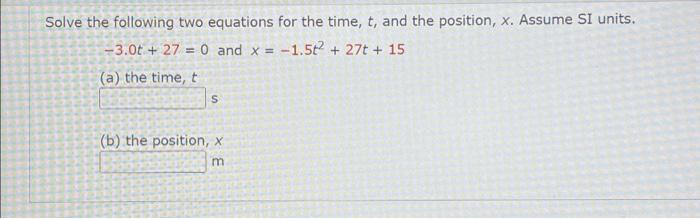# Question Solve the following two equations for the time, t, and the position, x. Assume SI units. -3.0t+27= 0 and x = -1.5t² + 27t + 15 (a) the time, t S (b) the position, x m Solve the following two equations for the time, t, and the position, x. Assume SI units. -3.0t+27= 0 and x = -1.5t² + 27t + 15 (a) the time, t S (b) the position, x m